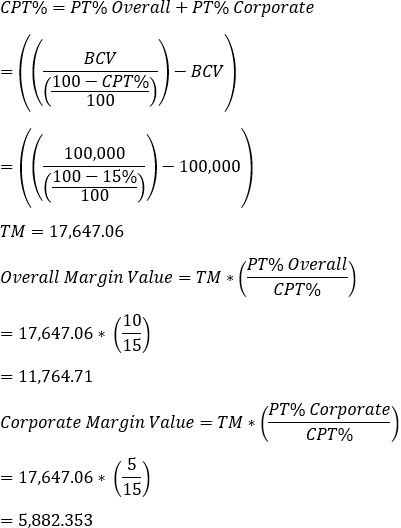## Project Summary – Combining Margin Calculations

Genesis and Express editions only.

In addition to adding a single margin type, Expert Estimation provides you with the ability to use both the Overall and Corporate Margin types simultaneously.

Note: Any costs associated with items using Override Margin are exempt from the general margin calculations.

#### ON TOTAL COSTS & ON TOTAL COSTS COMBINATION

When both Overall and Corporate margins are set to On Total Costs, both the margins will calculate using the Total Costs value of the project to generate their independent Margin values which are then combined to calculate Margin Total.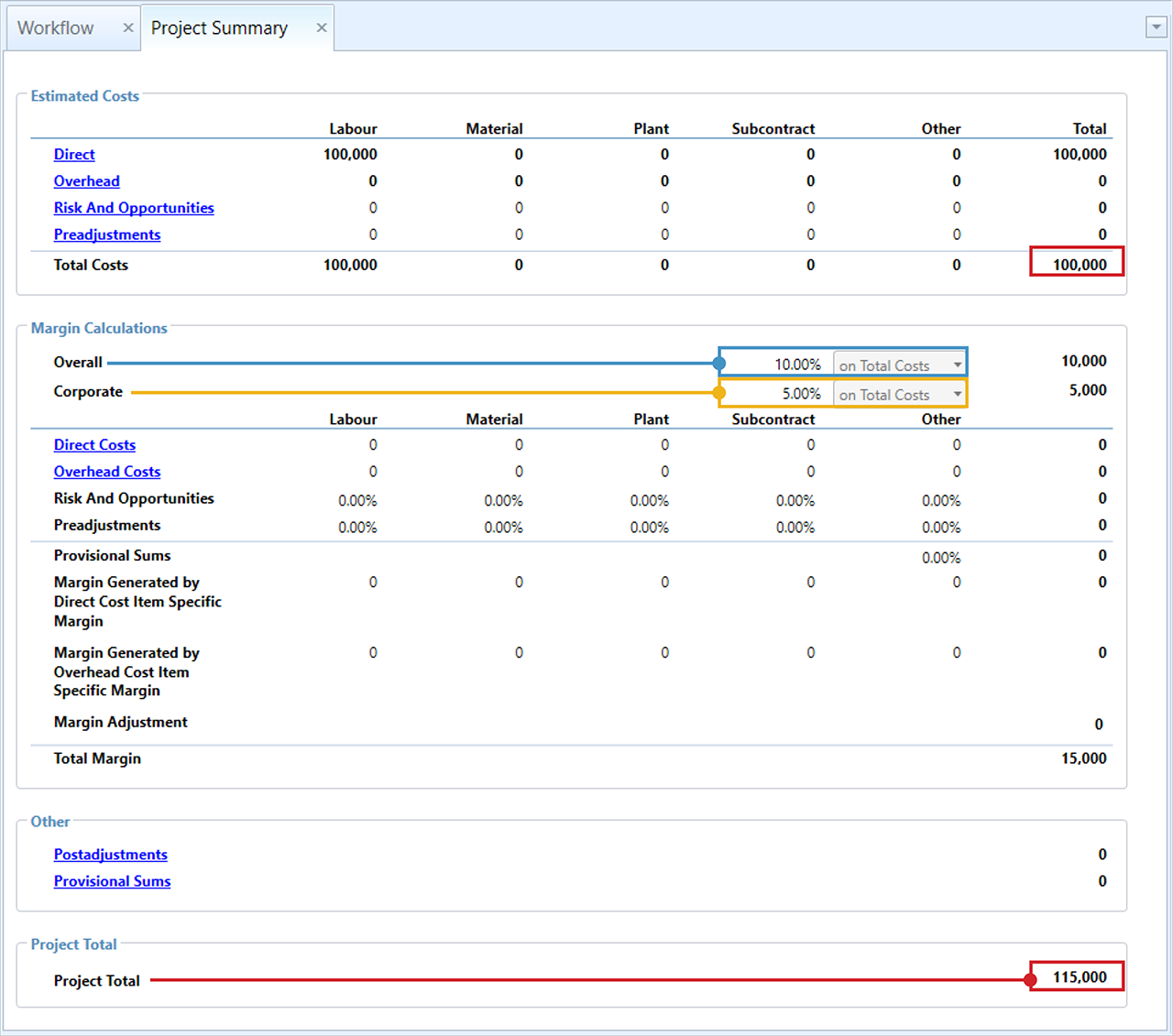Project Summary – Calculating margin combining ‘On Total Costs’

The formula below shows how the total margin is calculated when using On Total Costs for both Overall and Corporate margins.

• TC% Overall = The percentage specified on Total Costs for Overall margin = 10%
• TC% Corporate = The percentage specified on Total Costs for Corporate margin = 5%
• TCV = Total Cost Value = Direct Total + Overhead Total + Risk & Opportunities Total + Preadjustments Total = \$100,000
• TM = Total Margin generated = \$15,000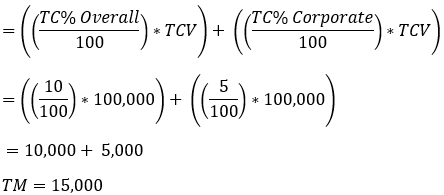#### ON TOTAL COST & OF PROJECT TOTAL COMBINATION

Margins using ‘of Project Total’ will be calculated after margins using ‘on Total Costs’. This means that when calculating the margin based on ‘of Project Total’, both Overall and Corporate margins will only include the other in their Base Contract Value portion of the function if the other’s value has been calculated using the ‘on Total Costs’ markup type.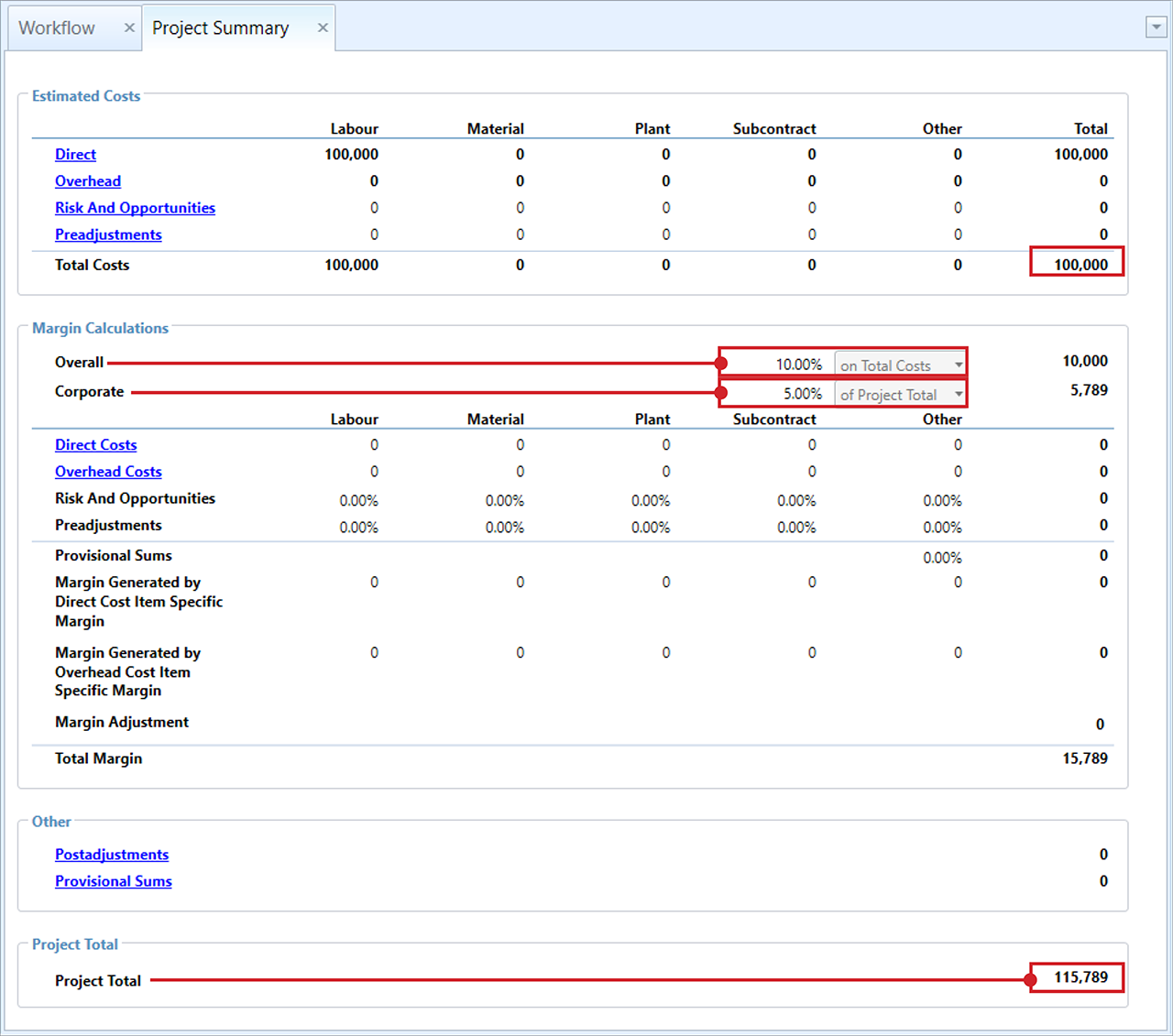Project Summary – Calculating margin ‘Of Project Total’ and ‘On Total Costs’

The formula below shows how the total margin that is calculated when using both On Total Costs and Of Project Total margin types.

• TC% = The percentage specified on Total Costs = 10%
• TCV = Total Cost Value = Direct Total + Overhead Total + Risk & Opportunities Total + Preadjustments Total = \$100,000
• PT% = The percentage specified of Project Total = 5%
• BCV = Base Contract Value = Total Cost Value + Direct Costs Margin + Overhead Costs Margin + Risk & Opportunities Margin + Preadjustments Margin + Provisional Sums Margin + Post margin Adjustment + Specific Margins + Margin Adjustment + Provisional Sums Total (if Calculation option has been set) = \$110,000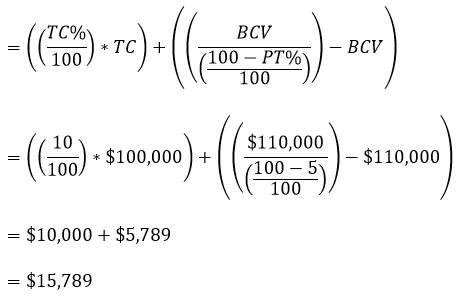#### OF PROJECT TOTAL & OF PROJECT TOTAL COMBINATION

When both Overall and Corporate Margins are set to ‘of Project Total’ the Total Margin generated is dependent on the calculation options set in the Project Properties – Calculations window.

##### INDIVIDUAL CALCULATION

When the calculation option in the Project Properties is set to Individually calculate Overall and Corporate Margins, each margin type will calculate a total margin, using the of Project Total calculation exclusive of the other margin type.

Once both margins have been calculated they are added together to generate the Total Margin.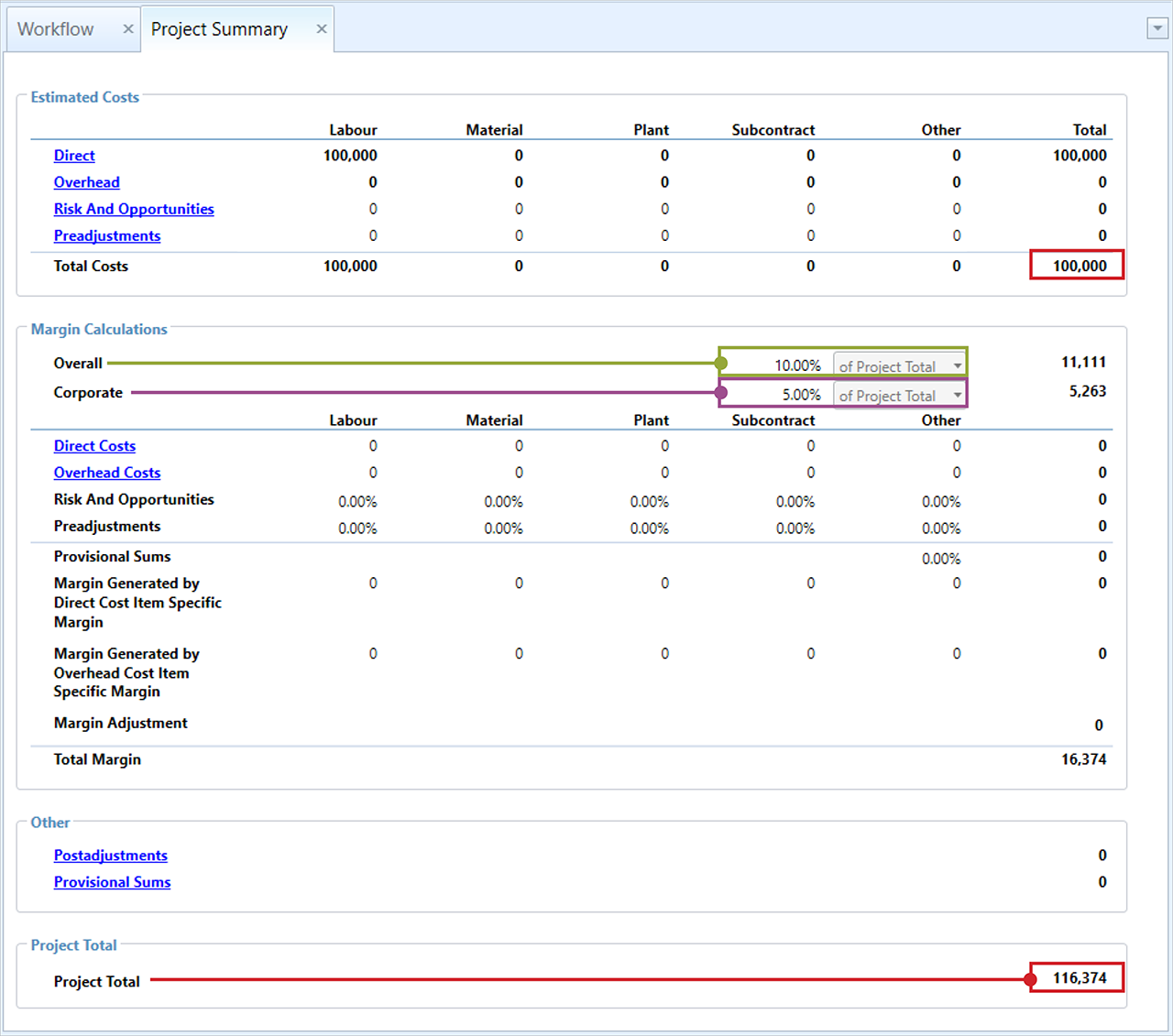Project Summary – Calculating margin ‘Of Project Total’ and Individually calculate Overall and Corporate Margins

The formula below shows how the total margin that is calculated when using Of Project Total for both Overall and Corporate margins with the option to individually calculate Overall and Corporate Margins is selected.

• PT% Overall = The percentage specified of Project Total for Overall margin = 10%
• PT% Corporate = The percentage specified of Project Total for Corporate margin = 5%
• BCV = Base Contract Value = Total Cost Value + Direct Costs Margin + Overhead Costs Margin + Risk & Opportunities Margin + Preadjustments Margin + Provisional Sums Margin + Post margin Adjustment + Specific Margins + Margin Adjustment + Provisional Sums Total (ifhas been set) = \$100,000
• TM = Total Margin = \$16,374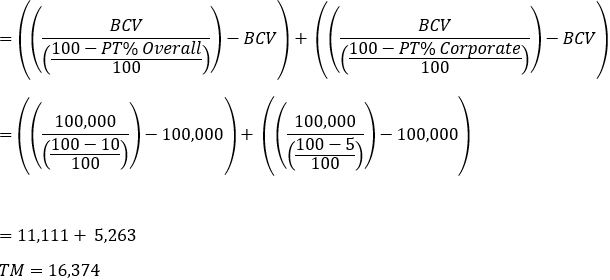##### COMBINED CALCULATION

When the calculation option is set to Combine Overall and Corporate Margin Calculations, the margin percentages for Overall and Corporate, will be added together to create a Combined Margin percentage.

The combined percentage is then used during the of Project Total calculation to generate the Total Margin for the project. Once the Total Margin is generated, the value is distributed between the two margins types proportionately.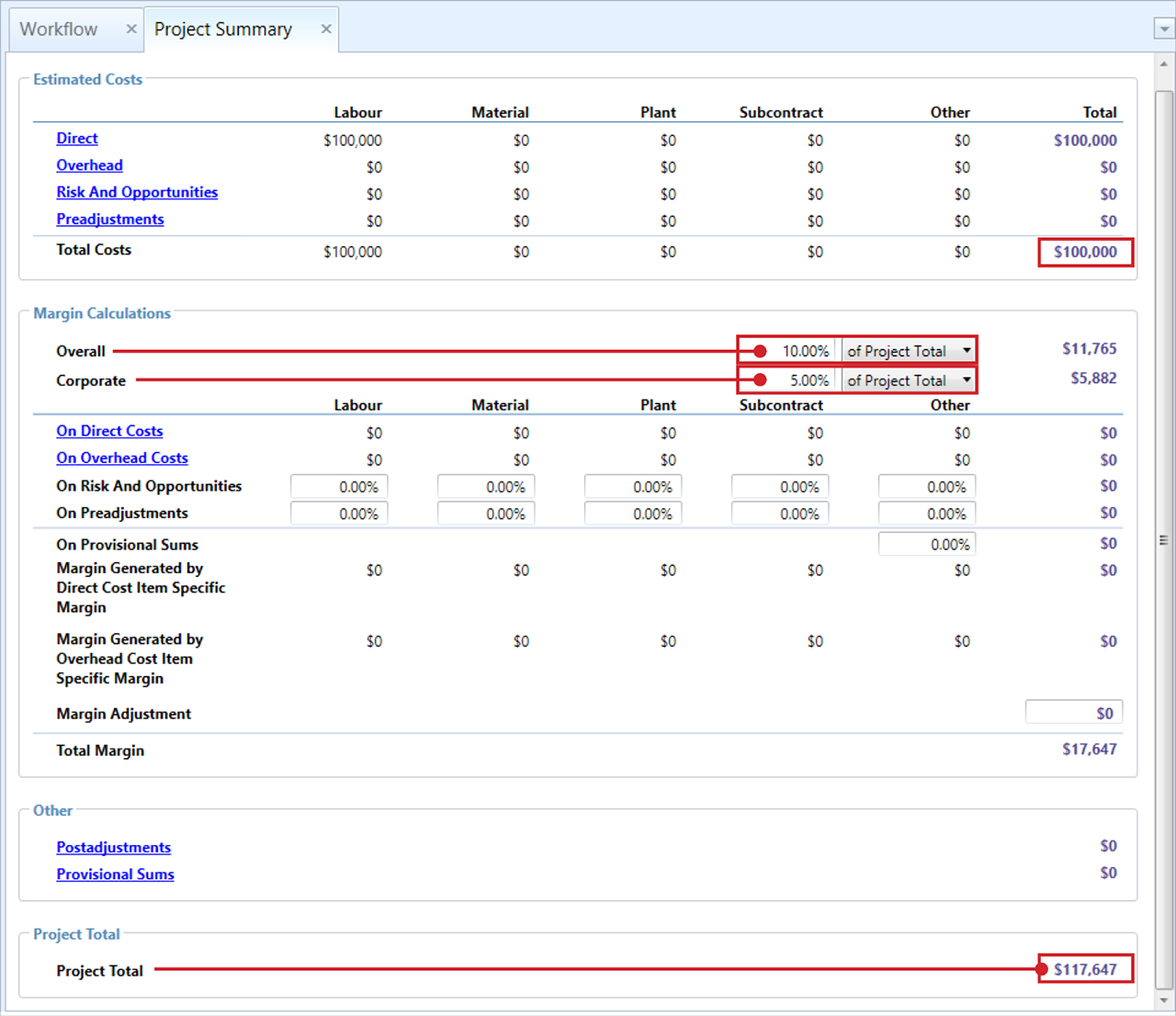Project Summary – Calculating margin ‘Of Project Total’ and Combine Overall and Corporate margin calculations

The formula below shows how the total margin that is calculated when using Of Project Total for both Overall and Corporate margins with the option to Combine Overall and Corporate Margin calculations selected.

• PT% Overall = The percentage specified of Project Total for Overall Margin = 10%
• PT% Corporate = The percentage specified of Project Total for Corporate Margin = 5%
• CPT% Corporate = The Combined Margin percent = 15%
• BCV = Base Contract Value = Total Cost Value + Direct Costs Margin + Overhead Costs Margin + Risk & Opportunities Margin + Preadjustments Margin + Provisional Sums Margin + Post margin Adjustment + Specific Margins + Margin Adjustment + Provisional Sums Total (if Calculation option has been set) = \$100,000
• TM = Total Margin = \$16,674